# Selina Concise Mathematics Class 6 ICSE Solutions Chapter 16 Percent (Percentage)

## Selina Concise Mathematics Class 6 ICSE Solutions Chapter 16 Percent (Percentage)

Selina Publishers Concise Mathematics Class 6 ICSE Solutions Chapter 16 Percent (Percentage)

### Percent Exercise 16A – Selina Concise Mathematics Class 6 ICSE Solutions

Question 1.
Express each of the following statements in the percentage form :
(i) 13 out of 20
(ii) 21 eggs out of 30 are good
Solution: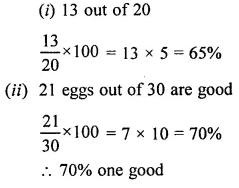Question 2.
Express the following fractions as percent :Solution:Question 3.
Express as percent:
(i) 0.10
(ii) 0.02
(iii) 0.7
(iv) 0.15
(v) 0.032
Solution:Question 4.
Convert into fractions in their lowest terms:
(i) 8%
(ii) 20%
(iii) 85%
(iv) 250%
(v) 12$$\frac { 1 }{ 2 }$$ %
Solution:Question 5.
Express as decimal fractions :
(i) 25%
(ii) 108%
(iii) 95%
(iv) 4.5%
(v) 29.2%
Solution: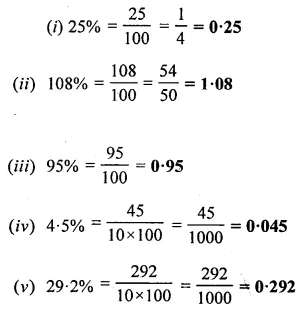Question 6.
Express each of the following natural numbers as percent :
(i) 7
(ii) 2
(iii) 19.5
(iv) 5.37
Solution: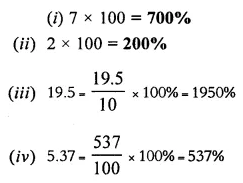### Percent Exercise 16B – Selina Concise Mathematics Class 6 ICSE Solutions

Question 1.
Express :
(i) Rs 5 as a percentage of Rs 25.
(ii) 80 paise as a percent of Rs 4.
(iii) 700 gm as a percentage of 2.8 kg.
(iv) 90 cm as a percent of 4.5 m.
Solution: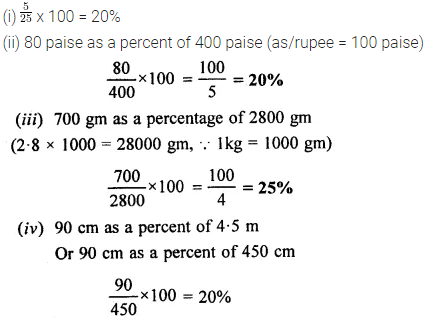Question 2.
Express the first quantity as a percent of the second :
(i)) 40 P, ₹ 2
(ii) 500 gm, 6 kg
(iii) 42 seconds, 6 minutes
Solution: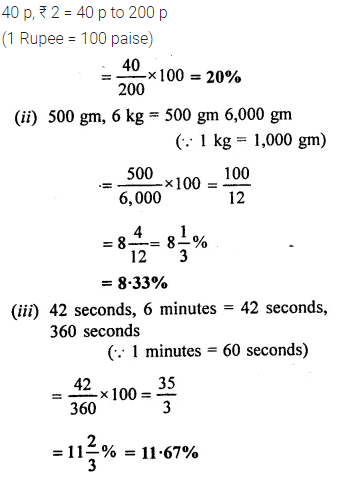Question 3.
Find the value of each of the following:
(i) 20% of ₹ 150
(ii) 90% of 130
(iii) 15% of 2 minutes
(iv) 7.5 % of 500 kg.
Solution: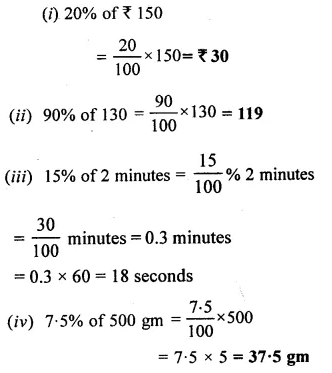Question 4.
If a man spends 70% of his income, what percent does he save ?
Solution: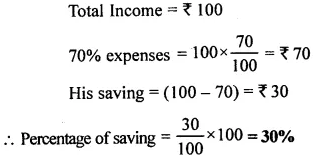Question 5.
A girl gets 65 marks out of 80. What percent marks did she get?
Solution: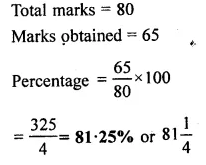Question 6.
A class contains 25 children, of which 6 are girls. What percentage of the class are the boys.
Solution: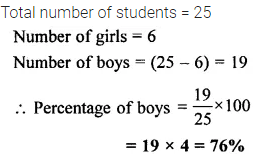Question 7.
A tin contains 20 litres of petrol. Due to leakage, 3 litres of petrol is lost. What percent is still present in the tin?
Solution:Question 8.
An alloy of copper and zinc contains 45% copper and the rest is zinc. Find the weight of zinc in 20 kg of the alloy.
Solution:Question 9.
A boy got 60 out of 80 in Hindi, 75 out of 100 in English and 65 out of 70 in Arithmetic. In which subject his percentage of marks the best ? Also, find his overall percentage.
Solution:Question 10.
In a camp, there were 500 soldiers. 60 more soldiers joined them. What percent of the earlier (original) number have joined the camp.
Solution:Question 11.
In a plot of ground of area 6000 sq. m, only 4500 sq. m is allowed for construction. What percent is to be left without construction?
Solution: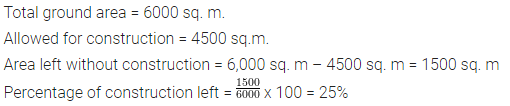Question 12.
Mr. Sharma has a monthly salary of ₹ 8,000. If he spends ₹ 6,400 every month; find :
(i) his monthly expenditure as percent.
(ii) his monthly savings as percent.
Solution: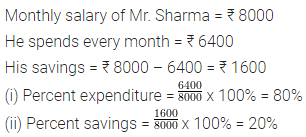Question 13.
The monthly salary of Rohit is ₹ 24,000. If his salary increases by 12%, find his new monthly salary
Solution: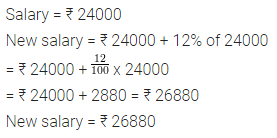Question 14.
In a sale, the price of an article is reduced by 30%. If the original price of the article is ₹ 1,800, find :
(i) the reduction in the price of the article
(ii) reduced price of the article.
Solution:Question 15.
Evaluate :
(i) 30% of 200 + 20% of 450 – 25% of 600
(ii) 10% of ₹ 450 – 12% of ₹ 500 + 8% of ₹ 500.
Solution:### Percent Exercise 16C – Selina Concise Mathematics Class 6 ICSE Solutions

Question 1.
The price of rice rises from Rs. 30 per kg to Rs. 36 per kg. Find the percentage rise in the price of rice.
Solution: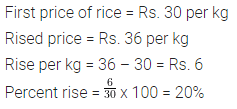Question 2.
The population of a small locality was 4000 in 1979 and 4500 in 1981, By what percent had the population increase ?
Solution:Question 3.
The price of a scooter was ₹ 8000 in 1975. It came down to ₹ 6000 in 1980. By what percent had the price of the scooter came down?
Solution: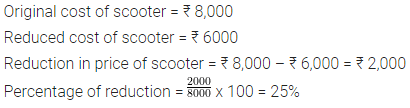Question 4.
Find the resulting quantity when :
(i) ₹ 400 is decreased by 8%.
(ii) 25 km is increased by 5%.
(iii) a speed of 600 km/h is increased by 12$$\frac { 1 }{ 2 }$$ %
(iv) there is 2.5% increase in a salary of ₹ 62, 500.
Solution: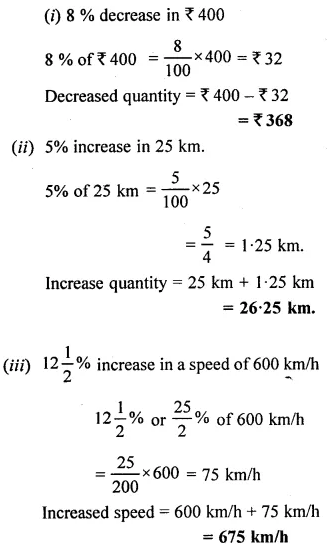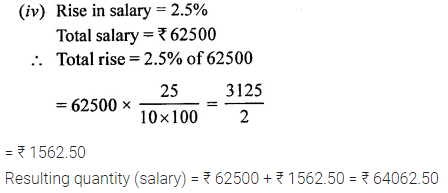Question 5.
The population of a village decreased by 12%. If the original population was 25,000, find the population after decrease?
Solution: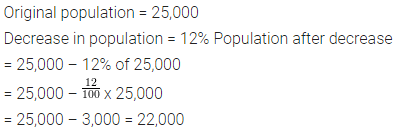Question 6.
Out of a salary of Rs. 13,500,1 keep 1/3 as savings. Of the remaining money, I spend 50% on food and 20% on house rent. How much do I spend on food and house rent?
Solution: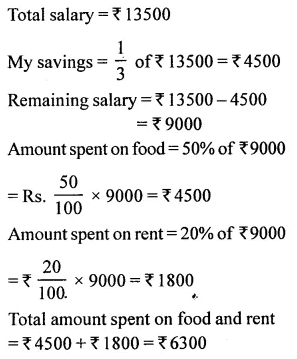Question 7.
A tank can hold 50 litres of water. At present, it is only 30% full. How many litres of water shall I put into the tank so that it becomes 50% full?
Solution: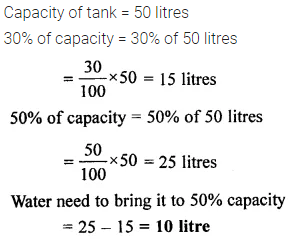Question 8.
In an election, there are a total of 80,000 voters and two candidates, A and B. 80% of the voters go to the polls out of which 60% vote for A. How many votes does B get.
Solution:Question 9.
70% of our body weight is made up of water. Find the weight of water in the body of a person whose body weight is 56 kg.
Solution: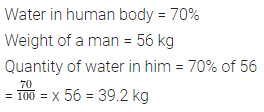Question 10.
Only one-fifth of water is available in liquid form. This limited amount of water is replenished and used by man recurrently. Express this information as percent, showing :
(i) water available in liquid form.
(ii) water available in frozen form.
Solution: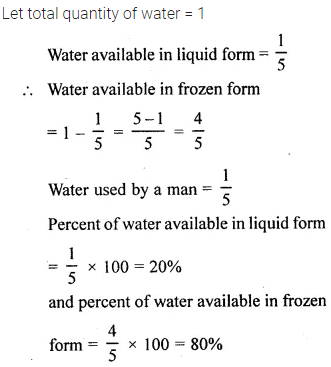Question 11.
By weight, 90% of tomato and 78% of potato is water. Find :
(i) the weight of water in 25 kg of tomato.
(ii) the total quantity, by weight, of water in 90 kg of potato and 30 kg of tomato
(iii) the weight of potato which contains 39 kg of water.
Solution: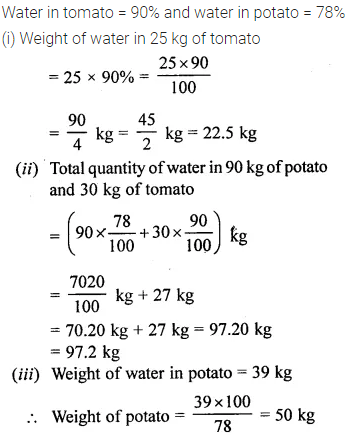### Percent Revision Exercise – Selina Concise Mathematics Class 6 ICSE Solutions

Question 1.
Rohit’s age is 12 years and Geeta’s age is 15 years. Express :
(i) Rohit’s age as a percent of Geeta’s age.
(ii) Geeta’s age as a percent of Rohit’s age.
Solution:Question 2.
A class has 30 boys and 20 girls. Find:
(i) the percentage of girls in the class
(ii) the percentage of boys in the class
(iii) percentage of number of boys as compared with number of girls.
Solution: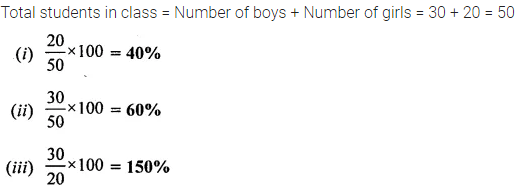Question 3.
Mrs. Sharma went to the market with ₹ 800 in her purse. When she returned to her home, ₹ 240 were still left in her purse. What percent of her money did she spend in the market?
Solution: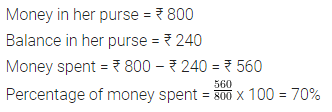Question 4.
In a mixture of two liquids A and B, 35% is liquid B. If the total quantity of the mixture is 20 kg, find the quantity of A, by weight.
Solution:Question 5.
A girl got 375 marks out of 500 in the first term examination, 560 marks out of 800 in the second term examination and 840 marks out of 1200 in the third term examination. Find:
(i) her percentage score in the first term examination.
(ii) her percentage score in the second term examination.
(iii) her percentage score in the third term examination.
(iv) the total marks secured in all the three examinations.
(v) the total marks scored in all the three examinations.
(vi) her percentage score on the whole in all the three examinations.
Solution: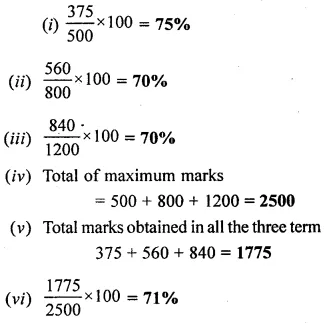Question 6.
Out of his monthly income of ₹ 2,500; a man spends ₹ 1,750. What percent of his income does he save every month?
Solution: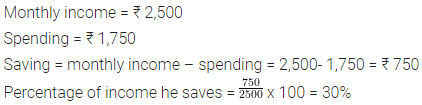Question 7.
Mr. Singh’s monthly salary is ₹ 15,000. This month he was promoted with an increment of ₹ 3,000 in his salary. Express his increment as a percent of his original salary.
Solution:Question 8.
(i) The price of an article increased from ₹ 16 to ₹ 20 ; find the percentage increase.
(ii) The price of an article decreased from Rs 20 to Rs 16 ; find the percentage decrease.
Solution: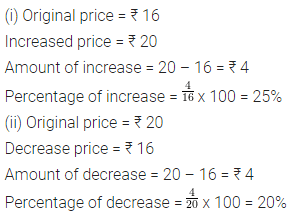Question 9.
(i)) The salary of a man is ₹ 7,200 per month, which is now increased by 8%. Find his new salary per month.
(ii) The salary of Mr. Sahni is ₹ 8,400 per month, which is now decreased by 8%. Find his new salary per month.
Solution: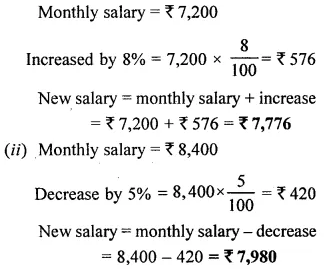Question 10.
Find the percentage change from the first quantity to the second :
(i) ₹ 80, ₹ 120
(ii) 75 kg, 60 kg
(iii) 50 cm, 45 cm
Solution:Question 11.
The original price of an article is ₹ 640. Find its new price when its price is :
(i) increased by 30%
(ii) decreased by 20%
Solution:Question 12.
Find the number that is :
(i) 50% more than 48
(ii) 30% less than 70
Solution:Question 13.
Evaluate :
(i) 8% of 900 – 12% of 750 + 20% of 165.
(ii) 70% of 70 + 90% of 90 – 120% of 120.
Solution:Question 14.
Approximately 97.3% water on the earth is not fit for drinking. Find :
(i) the percentage of water on the earth that is fit for drinking.
(ii) The total volume of water available in certain part of the earth where there is 21,600 m3 of drinking water.
Solution:Question 15.
Air is an important inexhaustible natural resource. It is essential for the survival of human beings, microbes, plants and animals. The following table shows the percentage of various gases in air.(i) In 800 m3 of air, calculate the approximate quantities of nitrogen, oxygen and other gases.
(ii) If a certain quantity (by volume) of air contains 4,200 litres of oxygen, find the total quantity of air taken and the amount of nitrogen in it.
Solution: